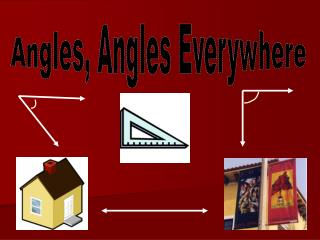DownloadDownload PresentationAngles, Angles Everywhere

# Angles, Angles Everywhere

Download Presentation## Angles, Angles Everywhere

- - - - - - - - - - - - - - - - - - - - - - - - - - - E N D - - - - - - - - - - - - - - - - - - - - - - - - - - -
##### Presentation Transcript

1. Angles, Angles Everywhere

2. Definition of an angle ~ Two rays with a common endpoint.

3. Right Angle A right angle is an angle that is 90°.

4. Acute Angles An acute angle is an angle less than 90° and larger than 0°. http://www.mathopenref.com/angleacute.html

5. Obtuse Angles An obtuse angle is an angle that is greater than 90° and less than 180°. http://www.mathopenref.com/angleobtuse.html

6. Straight Angles A straight angle is an angle that is 180°. It’s a straight line!

7. Practicing our angles • http://www.amblesideprimary.com/ambleweb/mentalmaths/protractor.html

8. Websites used • http://thesaurus.maths.org/mmkb/entry.html?action=entryById&id=775&msglang=da • http://www.homeschoolmath.net/teaching/g/measure_angles.php • http://images.google.com/imgres?imgurl=http://www.mathsisfun.com/images/rightangle.gif&imgrefurl=http://www.mathsisfun.com/rightangle.html&h=300&w=500&sz=4&tbnid=7sb6znW1M49TMM:&tbnh=78&tbnw=130&prev=/images%3Fq%3Dpicture%2Bof%2Bright%2Bangles%26um%3D1&start=1&sa=X&oi=images&ct=image&cd=1 • http://www.mathsisfun.com/geometry/images/straight-angles.gif